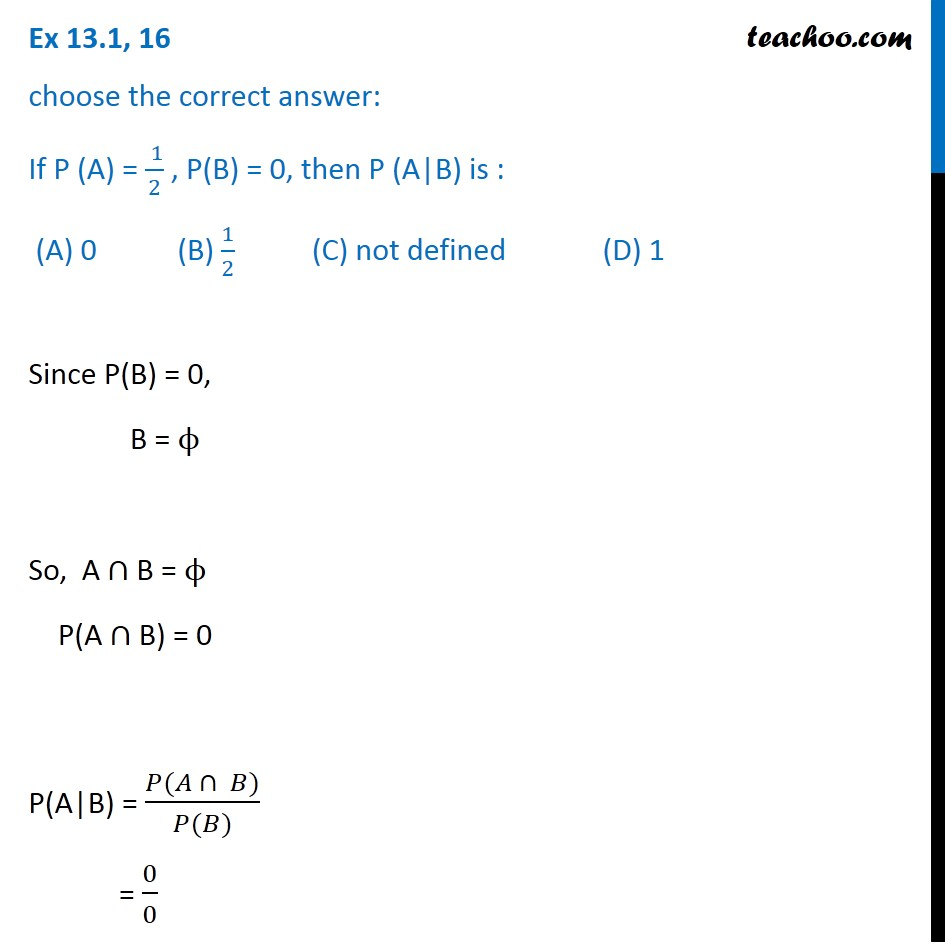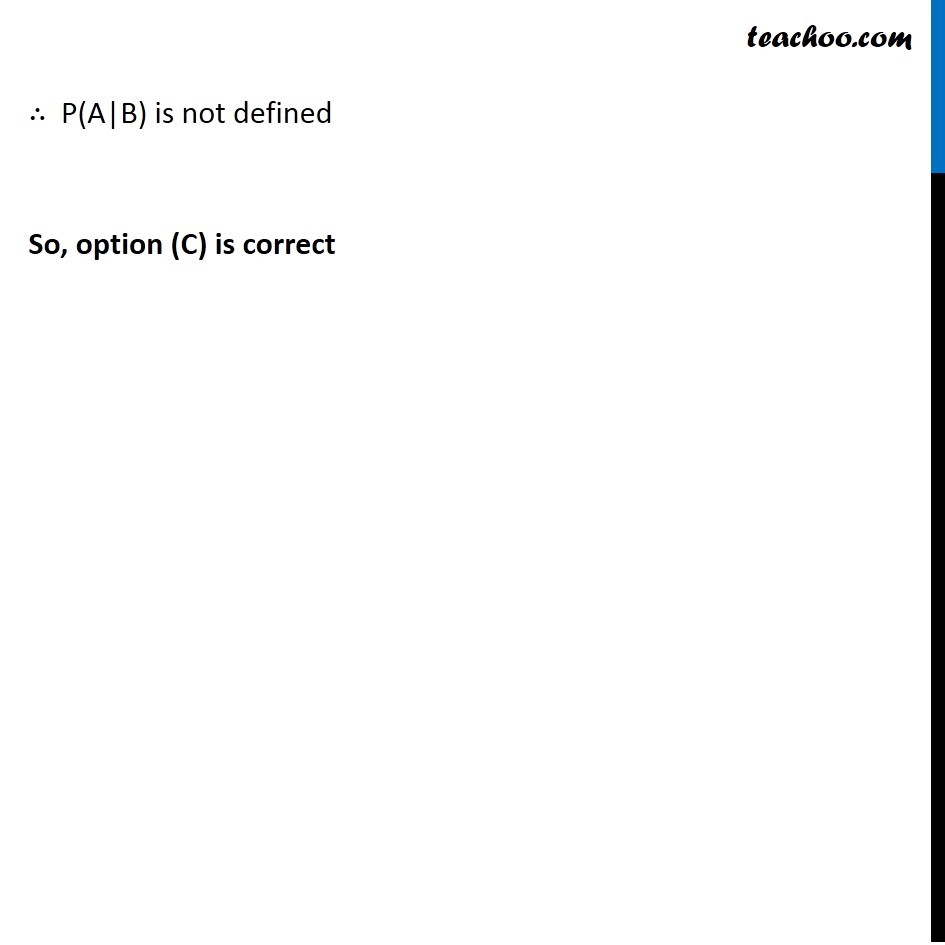Ex 13.1

Chapter 13 Class 12 Probability
Serial order wiseLearn in your speed, with individual attention - Teachoo Maths 1-on-1 Class

### Transcript

Ex 13.1, 16 Choose the correct answer: If P (A) = 1/2 , P(B) = 0, then P (A|B) is : (A) 0 (B) 1/2 (C) not defined (D) 1Since P(B) = 0, ∴ B = 𝝓 So, A ∩ B = ϕ P(A ∩ B) = 0 P(A|B) = (𝑃(𝐴 ∩ 𝐵))/(𝑃(𝐵)) = 𝟎/𝟎 ∴ P(A|B) is not defined So, Option (C) is correct